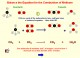Atoms, Symbols and Equations 4.0 Unique interactive multimedia Chemistry teaching software. SharewarePEEI 4.0.0.1 Solver of systems of partial differential equations. Freeware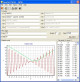EqPlot 1.3.22 EqPlot plots 2D graphs from complex equations. Shareware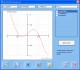DeadLine 2.36 Analyze, solve, and plot various mathematical equations. FreewareQuadEquations 2.0.1 QuadEquations facilitates the teaching and learning of quadratic equations. SharewareGraph550 0.5 Graph550 is a program to graph mathematical equations. FreewareInfinity 1.3.1 Innovative non-linear math software for high precision calculations. Try it now! Shareware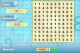Math Search 1.9.2 Solve the equations and look for the answers on the grid. Freeware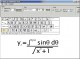Abacus Math Writer 4.0 For publishing Mathematical and Scientific equations. SharewareMath Squeeze 1.0.1 Exciting Math game for kids. Great for beginners to experts. Shareware